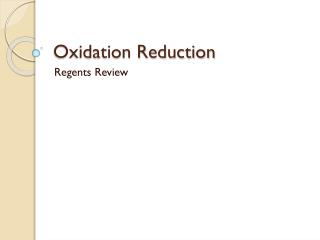Download PresentationOxidation Reduction

# Oxidation Reduction - PowerPoint PPT PresentationDownload Presentation## Oxidation Reduction

- - - - - - - - - - - - - - - - - - - - - - - - - - - E N D - - - - - - - - - - - - - - - - - - - - - - - - - - -
##### Presentation Transcript

1. Oxidation Reduction Regents Review

2. Zn(s) + Pb2+ (aq) → Zn2 + (aq) + Pb(s) In the completed external circuit, the electrons flow from: • A)    Pb(s) to Zn(s) • B)    Pb2+(aq) to Zn2+(aq) • C)    Zn(s) to Pb(s) • D)    Zn2+(aq) to Pb2+(aq) • Correct Answer: Option C - Zn(s) to Pb(s)

3. Correct Answer is flow of ions

4. All the metals listed above Mg in Reference Table J would be more readily oxidized (give up electrons

5. Correct answer: (2) Zn (s) ® Zn2+ (aq) + 2e-

6. Correct Answer:  (3) at the cathode in an electrolytic cell and the anode in a voltaic cell

8. Correct answer: In a voltaic cell, the reaction is spontaneous. In an electrolytic cell, it is nonspontaneous.

9. Correct answer: Al+3 + 3e- →  Al

10. (1) is the correct answer.  Reduction means the gain of an electron, which removes the positive charge from potassium (because an electron is negatively charged).

11. (3) the total number of electrons lost equals the number of electrons gained.

12. Given the redox reaction: Ni + Sn4+ → Ni2+ + Sn2+Which species has been oxidized? • Ni • Sn4+ • Ni2+ • Sn2+ • Correct Answer is Ni

13. In the reaction: 2H2(g) + O2(g) → 2H2O(g), the oxidizing agent is • H2 • O2 • H− • O2− • Correct answer is O2

14. The diagram shows a voltaic cell. The reaction occurs at 1 atmosphere and 298 K. When the switch is closed, what occurs? • Pb is oxidized and electrons flow to the Zn electrode. • Pb is reduced and electrons flow to the Zn electrode. • Zn is oxidized and electrons flow to the Pb electrode. • Zn is reduced and electrons flow to the Pb electrode. • Correct Answer is Choice 3

15. In the reaction: Pb + 2Ag+ → Pb2+ + 2Ag, the Ag+ is • reduced, and the oxidation number changes from +1 to 0 • reduced, and the oxidation number changes from +2 to 0 • oxidized, and the oxidation number changes from 0 to +1 • oxidized, and the oxidation number changes from +1 to 0 • Correct Answer is Choice 1

16. The diagram represents the electroplating of a metal fork with Ag(s). Which part of the electroplating is represented by the fork? • the anode, which is the negative electrode • the cathode, which is the negative electrode • the anode, which is the positive electrode • the cathode, which is the positive electrode • Correct Answer is Choice 2

17. The diagram represents the electroplating of a fork with Ag(s). Which equation represents the half-reaction that takes place at the fork? • Ag+ + NO3− → AgNO3 • AgNO3 → Ag+ + NO3− • Ag+ + e− → Ag(s) • Ag(s) → Ag+ + e− • Correct Answer is Choice 3

18. What is the oxidation number of iodine in NaIO3? • +1 • +5 • +3 • +6 • Correct Answer is +5

19. In the reaction: 2Mg + O2 → 2MgO, the magnesium is the • oxidizing agent and is reduced • oxidizing agent and is oxidized • reducing agent and is reduced • reducing agent and is oxidized • Correct Answer is Choice 4

20. The diagram represents a chemical cell at 298 K and 1 atmosphere. Which species represents the cathode? • Zn • Zn2+ • Cu • Cu2+ • Correct answer is Cu